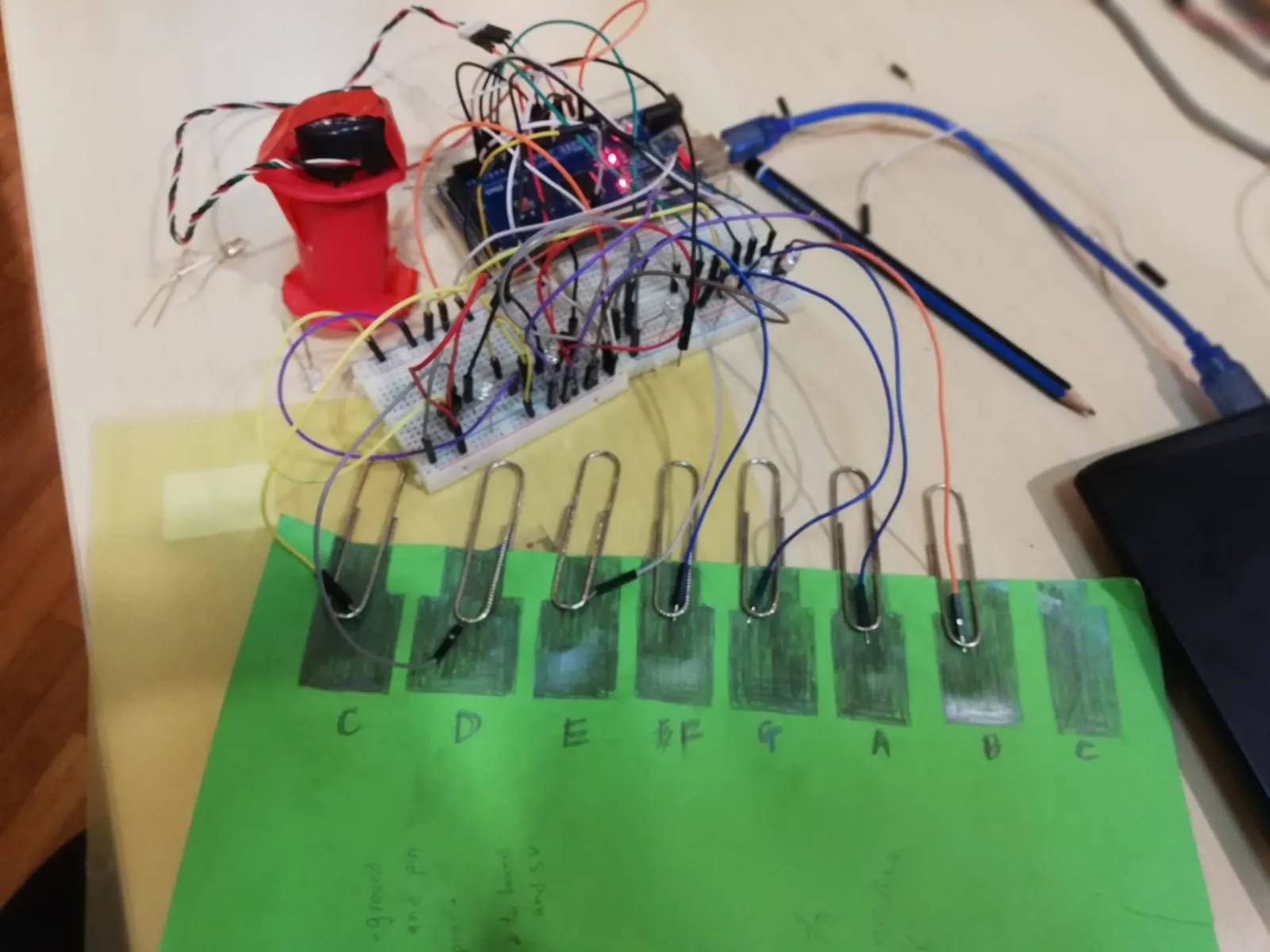Project tutorialTouch Sensing Paper Piano

A paper piano that relies on human touch for audio output using transistors.

• 1,495 views
• 1 comment
• 8 respects

Components and supplies

The concept of the touch sensing piano is simple. Voltage is fed through the collector of the NPN transistor. An open wire is also added at the collector for the hand the hold. As for the base of the transistor, it is connected to the paper piano. The emitter is connected to the LED which leads to ground, and an analog pin reads the voltage at the emitter as well. If a person touches the hand wire along with the piano key, the person connects the base and the collector together, hence providing an alternate path for the current to flow from the collector to the emitter, basically the hands are a form of a switch to turn on/off the signs. The analog pin detects the change in voltage, which then provides the audio output based on the pin of the piano key.

VIDEO OF PIANO:

for more info on transistors and their working principles - https://learn.sparkfun.com/tutorials/transistors/all

-things to note of:

the keys tend to conduct electricity on the same paper, hence, it is advisable to cut and stick key keys on a plastic sheet.

Code

piano code.Arduino
int pin = {A0,A1,A2,A8,A9,A10,A11}; // pin that reads voltage
int x;int y;int z;
#define speaker 3                     // speaker output pin
int volts = {analogRead (pin[x])};  //stores 10 readings of voltage for ONE pin
//number of samples for the average mean vaule is 10
int sum;int sum1;int average;       // aVERAGE VARIABLES
int store ;                    //stores AVERAGE reading of voltages of ALL pins

void setup() {

for (x = 0;x<=6; x++){
pinMode (pin[x], INPUT);
pinMode(speaker,OUTPUT);

Serial.begin (115200);}}

void loop() {
const int samples = 10;

for ( x = 0; x <= 6 ; x++){
for ( y = 0;y<= 9 ; y++){
analogRead (pin [x]);                    //read voltage at each pin
volts [y] = analogRead (pin [x]);          // store voltage of each pin in the array volts(up to 10 values as the array can only store 10 values)
sum = volts[y];                    // sum of all the votlage (10 values)
sum1 = sum += analogRead (pin [x]);  //add the previous analog reading to the current
average = sum1/samples;
store [x] = average;
Serial.println ("");
Serial.println (store );}}

const int EV =97;                     //value obtained through testing and observing how the voltage changes during serial.

if ( (store ) > EV){   // if average voltage for the first pin value stored in the array (pin A0) is larger than 97
tone (speaker,195.9977);}   // tone(pin,frequency) to play

else if ((store ) > EV ){
tone (speaker,220.0000);}

else if(store  > EV){
tone(speaker,246.9417);}

else if (store  > EV ){
tone ( speaker,261.6256);}

else if (store  > EV){
tone (speaker,293.6648);}

else if (store  > EV){
tone(speaker,329.6276);}

else if (store  > EV){
tone(speaker,523.2511);}

else {noTone(speaker);}} // prevents speaker from playing continuously.

Schematics

this is the circuit diagram for the piano. From the cover picture, it can be seen that is is complicated, hence, this circuit if for ONE PIANO KEY ONLY. The collector is connected to two things, which is a 5 volt rail, and a wire for the person's hand to hold to complete the circuit. The base is connected to the piano key, and the emitter is connected to the LED, which leads to a analog pi to read the change in voltage, and also to ground.

As for the buzzer, it is connected to a digital pin for its live wire so that it will only output audio when there is a change in voltage which is picked up by the analog pin, and ground obviously to ground.

It is highly recommended to create a three rails to simplify the circuit for all keys. These rails consists of a live rail for the 5v from the arduino, a ground rail as well as a rail for the hand wire.Paper Piano with Arduino

Project showcase by Ikhsan Ismail

• 10,596 views
• 68 respects

3D Printed Prosthetic Hand with Capacitive Touch Sensing

Project tutorial by Amal Mathew

• 3,560 views
• 23 respects

Copy of Paper Piano

Project tutorial by user4573

• 2,002 views
• 5 respects

Electronic Piano Keyboard With Preset Songs

Project tutorial by Lindsay Fox

• 77,975 views
• 150 respects

Arduino Tutorial : Mini Piano

Project tutorial by the lonely programmer

• 6,371 views